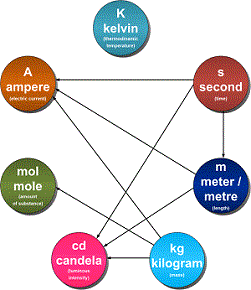# Topic: Units and Measurements (Test 1)

Topic: Units and Measurements
Q.1
Physical quantities are
A. quantities such as degrees, radians and steradians
B. quantities such as pounds, dollars and rupees
C. quantities such as length, mass, time, electric current, thermodynamic temperature, amount of substance, and luminous intensity
D. quantities such as kilos, pounds and gallons
Answer : Option C
Explaination / Solution:

A physical quantity is a physical property of a phenomenon, body, or substance, that can be quantified by measurement.A physical quantity can be expressed as the combination of a magnitude expressed by a number – usually a real number – and a unit. All these given above can be expressed as explained so these are physical quantity.

Workspace
Report
Q.2
Measurement of a physical quantity is essentially the
A. process of subdividing the physical quantity
B. process of comparing with a standard using an instrument
C. process of observing the physical quantity
D. process of taking readings on an instrument
Answer : Option B
Explaination / Solution:

The Measurement of a given quantity is essentially an act or result of comparison between a quantity whose magnitude (amount) is unknown, with a similar quantity whose magnitude (amount) is known, the latter quantity being called a Standard.

Workspace
Report
Q.3
Fundamental or base quantities are arbitrary. In SI system these are
A. length, mass, time, electric current, thermodynamic temperature, amount of substance, and luminous intensity
B. as length, mass, time, electric charge, thermodynamic temperature, amount of substance, and luminous intensity
C. length, force, time, electric current, thermodynamic temperature, amount of substance, and luminous intensity
D. length, mass, force, electric current, thermodynamic temperature, amount of substance, and luminous intensity
Answer : Option A
Explaination / Solution:

A base quantity is chosen and arbitrarily defined, rather than being derived from a combination of other physical quantities. The 7 base quantities are:

PHYSICAL QUANTITYBASE SI UNIT
Mass (m)Kilogram (Kg)
Length (ll)Metre (m)
Time (t)Second (s)
Current (II)Ampere (A)
Temperature (T)Kelvin (K)
Amount of sub. (n)Molar (mol)
Luminous Intensity (L)Candela (cd

Workspace
Report
Q.4
Unit for a fundamental physical quantity is
A. defined as best of various reference standards
B. the smallest measurable value of the physical quantity
C. reference standard for the physical quantity
D. defined as average various reference standards
Answer : Option C
Explaination / Solution:

Unit is the reference used as the standard measurement of a physical quantity. The unit in which the fundamental quantities are measured are called fundamental unit and the units used to measure derived quantities are called derived units.

Workspace
Report
Q.5
In SI system the fundamental units are
A. meter, Newton, second, ampere, Kelvin, mole and candela
B. meter, kilogram, second, ampere, Kelvin, mole and candela
C. meter, kilogram, second, ampere, Kelvin, mole and watt
D. meter, kilogram, second, coulomb, Kelvin, mole and candela, horse power
Answer : Option B
Explaination / Solution:

The SI base units and their physical quantities are the metre for measurement of length, the kilogram for mass, the second for time, the ampere for electric current, the kelvin for temperature, the candela for luminous intensity, and the mole for amount of substance.Workspace
Report
Q.6
Derived units
A. are units of physical quantity that can not be expressed as multiples of fundamental physical quantities
B. are units of physical quantity that can be expressed as a combination of fundamental physical quantities
C. are units of physical quantity that can be expressed as multiples of fundamental physical quantities
D. are units of physical quantity that cannot be expressed as a combination of fundamental physical quantities
Answer : Option B
Explaination / Solution:

Derived units are units which may be expressed in terms of base units by means of mathematical symbols of multiplication and division.

For example, the SI derived unit of area is the square metre (m​​​​​​2), and the SI derived unit of density is the kilogram per cubic metre (kg/m3 or kg m​​​​​​-3).

The names of SI units are written in lowercase.

Workspace
Report
Q.7
Joule is the SI unit of
A. acceleration
B. power
C. force
D. work
Answer : Option D
Explaination / Solution:

The joule (symbol J), is a derived unit of energy in the International System of Units. It is equal to the energy transferred to (or work done on) an object when a force of one newton acts on that object in the direction of its motion through a distance of one metre (1 newton metre or N⋅m).

Workspace
Report
Q.8
Newton is the SI unit of
A. acceleration
B. force
C. power
D. work
Answer : Option B
Explaination / Solution:

The newton is the SI unit for force; it is equal to the amount of net force required to accelerate a mass of one kilogram at a rate of one meter per second squared

Workspace
Report
Q.9
The least count of any measuring equipment is
A. the smallest reading on the instrument
B. the smallest quantity that can be measured using that instrument
C. the smallest range in any instrument
D. None of these
Answer : Option B
Explaination / Solution:

The smallest value that can be measured by the measuring instrument is called its least count. Measured values are good only up to this value. The least count error is the error associated with the resolution of the instrument.

Workspace
Report
Q.10
Resolution is
A. the smallest amount of input signal change that the instrument can detect reliably
B. a measure of the bias in the instrument
C. a measure of the systematic errors
D. None of these
Answer : Option A
Explaination / Solution:

Resolution is the smallest amount of input signal change that the instrument can detect reliably. This term is determined by the instrument noise (either circuit or quantization noise).

Workspace
Report